# Buffalo shootings paper published

My article examining spatial shifts in shootings in Buffalo pre/post Covid, in collaboration with several of my Buffalo colleagues, is now published in the Journal of Experimental Criminology (Drake et al., 2022).

If you do not have access to that journal, you can always just email, or check out the open access pre-print. About the only difference is a supplement we added in response to reviewers, including maps of different grid cell areas, here is a hex grid version of the changes:The idea behind this paper was to see if given the dramatic increase in shootings in Buffalo after Covid started (Kim & Phillips, 2021), they about doubled (similar to NYC), did spatial hot spots change? The answer is basically no (and I did a similar analysis in NYC as well).

While other papers have pointed out that crime increases disproportionately impact minority communities (Schleimer et al., 2022), which is true, it stands to be very specific what the differences in my work and this are saying. Imagine we have two neighborhoods:

``````Neighborhood A, Disadvantaged/Minority, Pre 100 crimes, Post 200 crimes
Neighborhood B,    Advantaged/Majority, Pre   1 crimes, Post   2 crimes
``````

The work that I have done has pointed to these increases due to Covid being that relative proportions/rates are about the same (shootings ~doubled in both Buffalo/NYC). And that doubling was spread out pretty much everywhere. It is certainly reasonable to interpret this as an increased burden in minority communities, even if proportional trends are the same everywhere.

This proportional change tends to occur when crime declines as well (e.g. Weisburd & Zastrow, 2022; Wheeler et al., 2016). And this just speaks to the stickiness of hot spots of crime. Even with large macro changes in temporal crime trends, crime hot spots are very durable over time. So I really think it makes the most sense for police departments to have long term strategies to deal with hot spots of crime, and they don’t need to change targeted areas very often.

# Spatial analysis of NYC Shootings using the SPPT

As a follow up to my prior post on spatial sample size recommendations for the SPPT test, I figured I would show an actual analysis of spatial changes in crime. I’ve previously written about how NYC shootings appear to be going up by a similar amount in each precinct. We can do a similar analysis, but at smaller geographic spatial units, to see if that holds true for everywhere.

The data and R code to follow along can be downloaded here. But I will copy-paste below to walk you through.

So first I load in the libraries I will be using and set my working directory:

``````###################################################
library(sppt)
library(sp)
library(raster)
library(rgdal)
library(rgeos)

my_dir <- 'C:\\Users\\andre\\OneDrive\\Desktop\\NYC_Shootings_SPPT'
setwd(my_dir)
###################################################
``````

Now we just need to do alittle data prep for the NYC data. Concat the old and new files, convert the data fields for some of the info, and do some date manipulation. I choose the pre/post date here March 1st 2020, but also note we had the Floyd protests not to long after (so calling these Covid vs protest increases is pretty much confounded).

``````###################################################
# Read in the shooting data

# Just one column off
print( cbind(names(old_shoot), names(new_shoot)) )
names(new_shoot) <- names(old_shoot)
shooting <- rbind(old_shoot,new_shoot)

# I need to conver the coordinates to numeric fields
# and the dates to a date field

coord_fields <- c('X_COORD_CD','Y_COORD_CD')
for (c in coord_fields){
shooting[,c] <- as.numeric(gsub(",","",shooting[,c])) #replacing commas in 2018 data
}

# How many per year to check no funny business
table(substring(shooting\$OCCUR_DATE,7,10))

# Making a datetime variable in R
shooting\$OCCUR_DATE <- as.Date(shooting\$OCCUR_DATE, format = "%m/%d/%Y", tz = "America/New_York")

# Making a post date to split after Covid started
begin_date <- as.Date('03/01/2020', format="%m/%d/%Y")
shooting\$Pre <- ifelse(shooting\$OCCUR_DATE < begin_date,1,0)

#There is no missing data
summary(shooting)
###################################################
``````

Next I read in a shapefile of the census tracts for NYC. (Pro-tip for NYC GIS data, I like to use Bytes of the Big Apple where available.) The interior has a few dongles (probably for here should have started with a borough outline file), so I do a tiny buffer to get rid of those interior dongles, and then smooth the polygon slightly. To check and make sure my crime data lines up, I superimpose with a tiny dot map — this is also a great/simple way to see the overall shooting density without the hassle of other types of hot spot maps.

``````###################################################
# Read in the census tract data

summary(nyc_ct)
plot(nyc_ct)
nrow(nyc_ct) #2165 tracts

# Dissolve to a citywide file
nyc_ct\$const <- 1
nyc_outline <- gUnaryUnion(nyc_ct, id = nyc_ct\$const)
plot(nyc_outline)

# Area in square feet
total_area <- area(nyc_outline)
# 8423930027

# Turning crimes into spatial point data frame
coordinates(shooting) <- coord_fields
crs(shooting) <- crs(nyc_ct)

# This gets rid of a few dongles in the interior
nyc_buff <- gBuffer(nyc_outline,1,byid=FALSE)
nyc_simpler <- gSimplify(nyc_buff, 500, topologyPreserve=FALSE)

# Checking to make sure everything lines up
png('NYC_Shootings.png',units='in',res=1000,height=6,width=6,type='cairo')
plot(nyc_simpler)
points(coordinates(shooting),pch='.')
dev.off()
###################################################
``````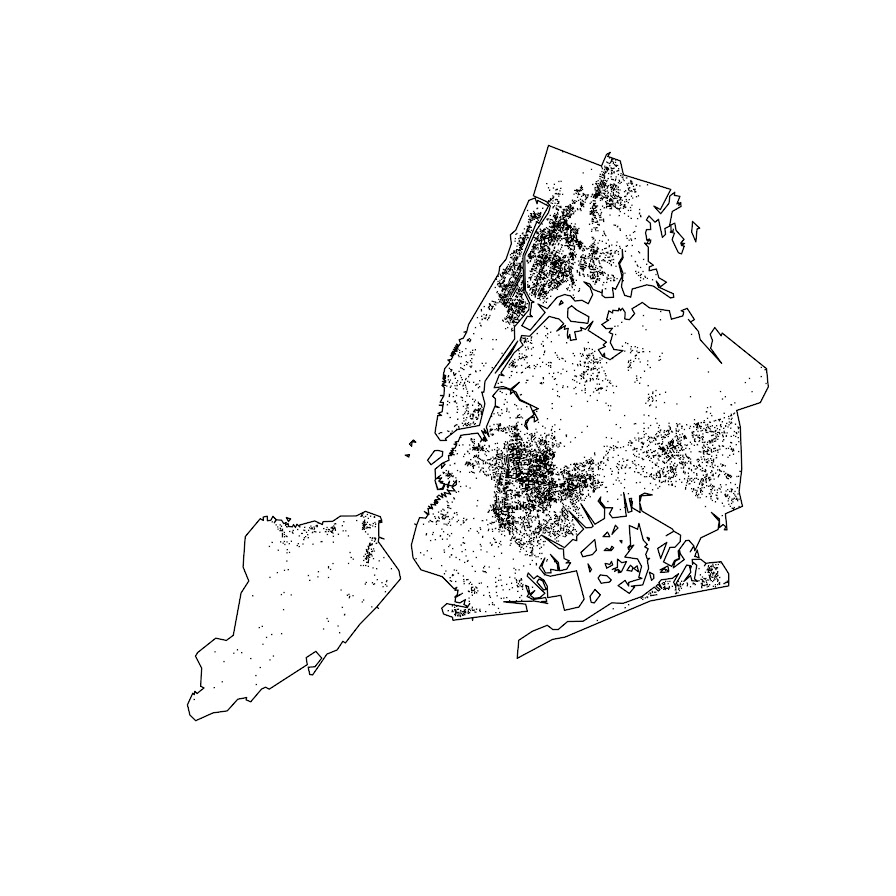The next part I created a function to generate a nice grid over an outline area of your choice to do the SPPT analysis. What this does is generates the regular grid, turns it from a raster to a vector polygon format, and then filters out polygons with 0 overlapping crimes (so in the subsequent SPPT test these areas will all be 0% vs 0%, so not much point in checking them for differences over time!).

You can see the logic from the prior blog post, if I want to use the area with power to detect big changes, I want `N*0.85`. Since I am comparing data over 10 years compared to 1+ years, they are big differences, so I treat N here as 1.5 times the newer dataset, which ends up being around a suggested 3,141 spatial units. Given the area for the overall NYC, this translates to grid cells that are about 1600 by 1600 feet. Once I select out all the 0 grid cells, there only ends up being a total of 1,655 grid cells for the final SPPT analysis.

``````###################################################
# Function to create sppt grid over areas with
# Observed crimes

grid_crimes <- function(outline,crimes,size){
# First creating a raster given the outline extent
base_raster <- raster(ext = extent(outline), res=size)
projection(base_raster) <- crs(outline)
# Getting the coverage for a grid cell over the city area
# Turning into a polygon
base_poly <- rasterToPolygons(base_raster,dissolve=FALSE)
xy_df <- as.data.frame(base_raster,long=T,xy=T)
base_poly\$x <- xy_df\$x
base_poly\$y <- xy_df\$y
base_poly\$poly_id <- 1:nrow(base_poly)
# May also want to select based on layer value
# sel_poly <- base_poly[base_poly\$layer > 0.05,]
# means the grid cell has more than 5% in the outline area
# Selecting only grid cells with an observed crime
ov_crime <- over(crimes,base_poly)
any_crime <- unique(ov_crime\$poly_id)
sub_poly <- base_poly[base_poly\$poly_id %in% any_crime,]
# Redo the id
sub_poly\$poly_id <- 1:nrow(sub_poly)
return(sub_poly)
}

# Calculating suggested sample size
total_counts <- as.data.frame(table(shooting\$Pre))
print(total_counts)

# Lets go with the pre-total times 1.5
total_n <- total_counts\$Freq*1.5

# Figure out the total number of grid cells
# Given the total area
side <- sqrt( total_area/total_n )
print(side)
# 1637, lets just round down to 1600

poly_cells <- grid_crimes(nyc_simpler,shooting,1600)
print(nrow(poly_cells)) #1655

png('NYC_GridCells.png',units='in',res=1000,height=6,width=6,type='cairo')
plot(nyc_simpler)
dev.off()
###################################################
``````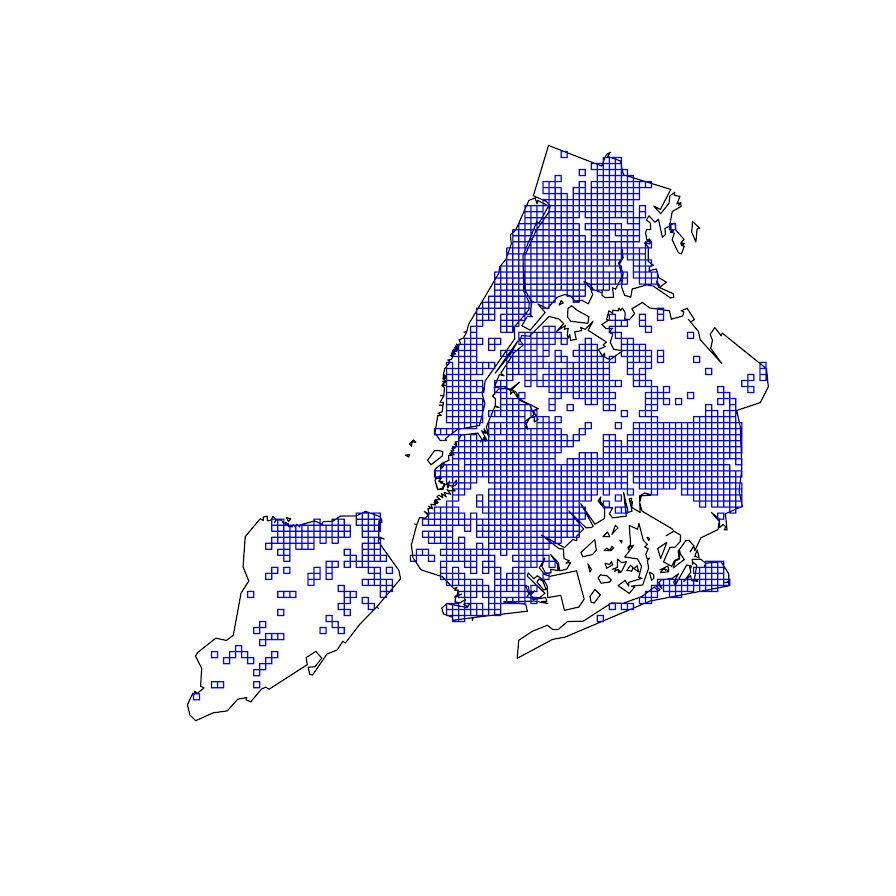Next part is to split the data into pre/post, and do the SPPT analysis. Here I use all the defaults, the Chi-square test for proportional differences, along with a correction for multiple comparisons. Without the multiple comparison correction, we have a total of 174 grid cells that have a p-value < 0.05 for the differences in proportions for an S index of around 89%. With the multiple comparison correction though, the majority of those p-values are adjusted to be above 0.05, and only 25 remain afterwards (98% S-index). You can see in the screenshot that all of those significant differences are increases in proportions from the pre to post. While a few are 0 shootings to a handful of shootings (suggesting diffusion), the majority are areas that had multiple shootings in the historical data, they are just at a higher intensity now.

``````###################################################
# Now lets do the sppt analysis

split_shoot <- split(shooting,shooting\$Pre)
pre <- split_shoot\$`1`
post <- split_shoot\$`0`

library(dplyr)
sppt_diff <- sppt_diff(pre, post, poly_cells)
summary(sppt_diff)

sum(sppt_diff\$p.value < 0.05) #174, around 89% similarity
sum(sppt_diff\$p.adjusted  < 0.05) #25, 98% similarity

# Lets select out the increases/decreases
# And just map those

sppt_sig <- sppt_diff[sig,]
head(sppt_sig,25) # to check out all increases
###################################################
``````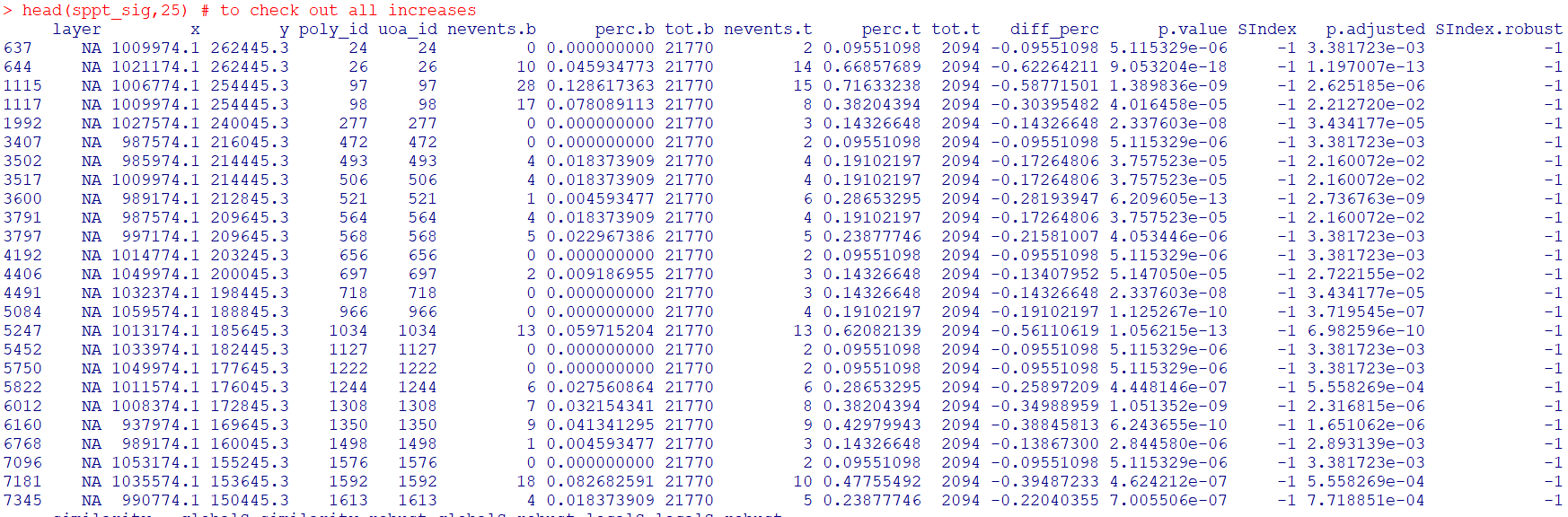The table is not all that helpful though for really digging into patterns, we need to map out the differences. The first here is a map showing the significant grid cells. They are somewhat tiny though, so you have to kind of look close to see where they are. The second map uses proportional circles to the percent difference (so bigger circles show larger increases). I am too lazy to do a legend/scale, but see my prior post on a hexbin map, or the sp website in the comments.

``````###################################################
# Making a map
png('NYC_SigCells.png',units='in',res=1000,height=6,width=6,type='cairo')
plot(nyc_simpler,lwd=1.5)
dev.off()

circ_sizes <- sqrt(-sppt_sig\$diff_perc)*3

png('NYC_SigCircles.png',units='in',res=1000,height=6,width=6,type='cairo')
plot(nyc_simpler,lwd=1.5)
points(coordinates(sppt_sig),pch=21,cex=circ_sizes,bg='red')
dev.off()

# check out https://edzer.github.io/sp/
# For nicer maps/legends/etc.
###################################################
``````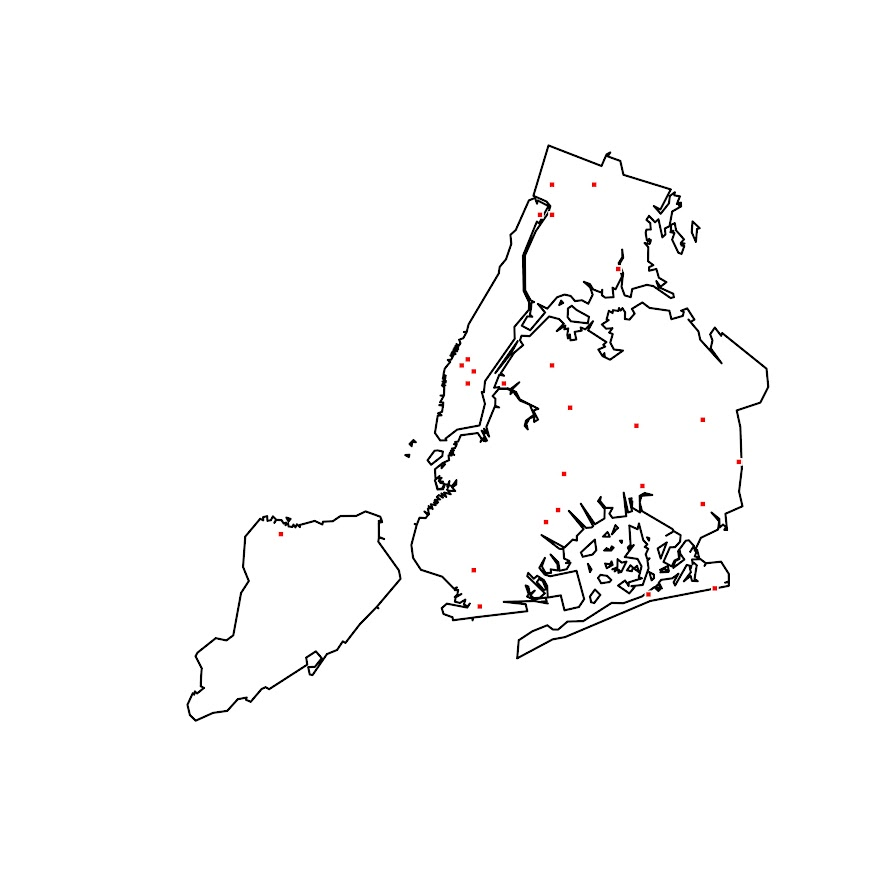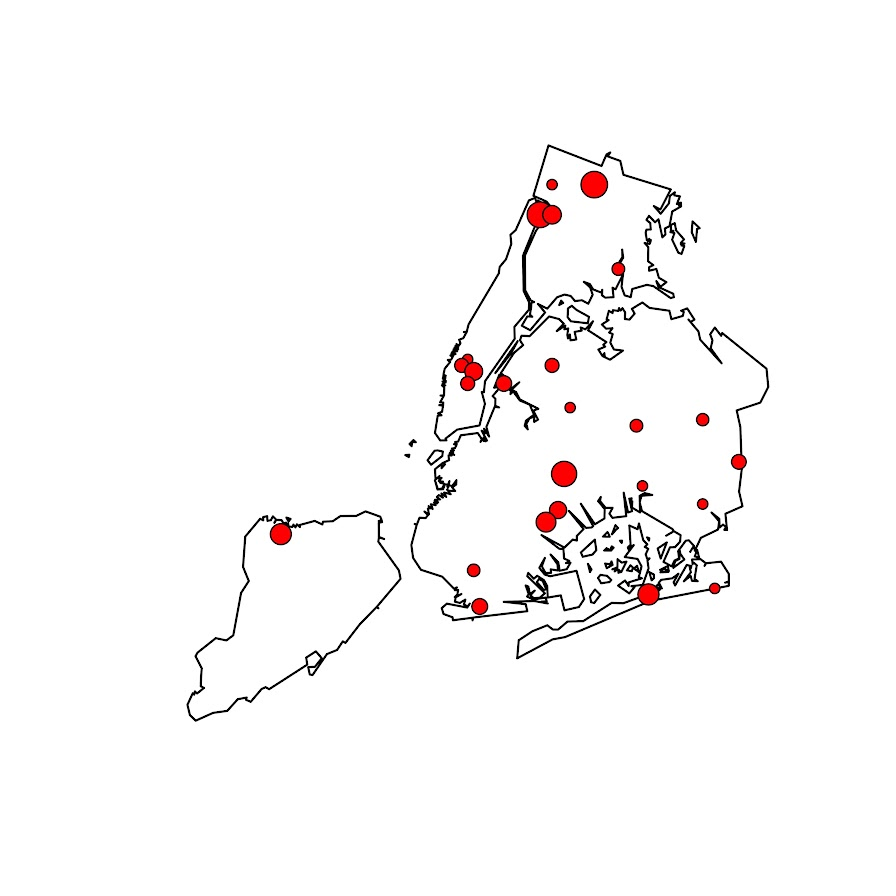So the increases appear pretty spread out. We have a few notable ones that made the news right in the thick of things in Manhattan, but there are examples of grid cells that increased scattered all over the boroughs. I am not going to the trouble here, but if I were a crime analyst working on this, I would export this to a format where I could zoom into the local areas and drill down into the specific incidents. You can do that either in ArcGIS, or more directly in R by creating a leaflet map.

So if folks have any better ideas for testing out crime increases I am all ears. At some point will give the R package sparr a try. (Here you could treat pre as the controls and post as the cases.) I am not a real big fan of over interpreting changes in kernel density estimates though (they can be quite noisy, and heavily influenced by the bandwidth), so I do like the SPPT analysis by default (but it swaps out a different problem with choosing a reasonable grid cell size).

# Spatial sample size suggestions for SPPT analysis

I’ve reviewed several papers recently that use Martin Andresen’s Spatial Point Pattern Test (Andresen, 2016). I have been critical of these papers, as I think they are using too small of samples to be reasonable. So here in this blog post I will lay out spatial sample size recommendations. Or more specifically if you have N crimes, advice about how you can conduct the SPPT test in S spatial units of analysis.

Long story short, if you have N crimes, I think you should either use `0.85*N = S` spatial units of analysis at the high end, but can only detect very large changes. To be well powered to detect smaller changes between the two distributions, use `0.45*N = S`. That is, if you have two crime samples you want to compare, and the smaller sample has 1000 crimes, the largest spatial sample size I would recommend is 850 units, but I think 450 units is better.

For those not familiar with the SPPT technique, it compares the proportion of events falling inside a common area (e.g. police beats, census block groups, etc.) between two patterns. So for example in my work I compared the proportion of violent crime and the proportion of SQF in New York City (Wheeler et al., 2018). I think it makes sense as a gross monitoring metric for PDs this way (say for those doing DDACTS, swap out pedestrian stops with traffic stops), so you can say things like `area A had a much lower proportion of crimes than stops, so we should emphasize people do fewer stops in A overall`.

If you are a PD, you may already know the spatial units you want to use for monitoring purposes (say for each police sector or precinct). In that case, you want the power analysis to help guide you for how large a sample you need to effectively know how often you can update the estimates (e.g. you may only have enough traffic stops and violent crimes to do the estimates on a quarterly basis, not a monthly one) Many academic papers though are just generally theory testing, so don’t have an a priori spatial unit of analysis chosen. (But they do have two samples, e.g. a historical sample of 2000 shootings and a current sample of 1000 shootings.) See Martin’s site for a list of prior papers using the SPPT to see it in action.

I’ve reviewed several papers that examine these proportion changes using the SPPT at very tiny spatial units of analysis, such as street segments. They also happen to have very tiny numbers of overall crimes, and then break the crimes into subsequent subsets. For example reviewed a paper that had around 100 crimes in each subset of interest, and had around 20,000 street segments. I totally get wanting to examine micro place crime patterns – but the SPPT is not well suited for this I am afraid.

Ultimately if you chunk up the total number of crimes into smaller and smaller areas, you will have less statistical power to uncover differences. With very tiny total crime counts, you will be basically only identifying differences between areas that go from 0 to 1 or 0 to 2 etc. It also becomes much more important to control for multiple comparisons when using a large number of spatial units. In general this technique is not going to work out well for micro units of analysis, it will only really work out for larger spatial units IMO. But here I will give my best advice about how small you can reasonably go for the analysis.

# Power analysis logic

There are quite a few different ways people have suggested to determine the spatial sample for areas when conducting quadrat analysis (e.g. when you make your own spatial areas). So one rule of thumb is to use `2*A/N`, where A is the area of the study and N is the total number of events (Paez, 2021).

Using the SPPT test itself, Malleson et al. (2019) identify the area at which the spatial pattern exhibits the highest similarity index with itself using a resampling approach. Ramos et al. (2021) look at the smallest spatial unit at which the crime patterns within that unit show spatial randomness.

So those later two take an error metric based approach (the spatial unit of analysis likely to result in the miminal amount of error, with error defined different ways). I take a different approach here – power analysis. We want to compare to spatial point patterns for proportional differences, how can we construct the test to be reasonably powered to identify differences we want to detect?

I do not have a perfect way to do this power analysis, but here is my logic. Crime patterns are often slightly overdispersed, so here I assume if you split up say 1000 crimes into 600 areas, it will have an NB2 distribution with a mean of `1000/600 = 1.67` and an overdispersion parameter of 2. (I assume this parameter to be 2 for various reasons, based on prior analysis of crime patterns, and that 2 tends to be in the general ballpark for the amount of overdispersion.) So now we want to see what it would take to go from a hot spot of crime, say the 98th percentile of this distribution to the median 50th percentile.

So in R code, to translate the NB2 mean/dispersion to N & P notation results in N & P parameters of `1.0416667` and `0.3846154` respectively:

``````trans_np <- function(mu,disp){
a <- disp
x <- mu^2/(1 - mu + a*mu)
p <- x/(x + mu)
n <- (mu*p)/(1-p)
return(c(n,p))
}

# Mean 1000/600 and dispersion of 2
nb_dis <- trans_np(1000/600,2)``````

Now we want to see what the counts are to go from the 98th to the 50th percentile of this distribution:

``crime_counts <- qnbinom(c(0.98,0.5), size=nb_dis, prob=nb_dis)``

And this gives us a result of ` 8 1` in the `crime_counts` object. So a hot spot place in this scenario will have around 8 crimes, and the median will be around 1 crime in our hypothetical areas. So we can translate these to percentages, and then feed them into R’s `power.prop.test` function:

``````crime_prop <- crime_counts/1000
power.prop.test(n = 1000, p1 = crime_prop, p2 = crime_prop)``````

And this gives us a result of:

``````     Two-sample comparison of proportions power calculation

n = 1000
p1 = 0.008
p2 = 0.001
sig.level = 0.05
power = 0.6477139
alternative = two.sided

NOTE: n is number in *each* group``````

Note that this is for one N estimate, and assumes that N will be the same for each proportion. In practice for the SPPT test this is not true, oftentimes we have two crime samples (or crime vs police actions like stops), which have very different total baseline N’s. (It is part of the reason the test is useful, it doesn’t make so much sense in that case to compare densities as it does proportions.) So subsequently when we do these estimates, we should either take the average of the total number of crimes we have in our two point patterns for SPPT (if they are close to the same size), or the minimum number of events if they are very disparate. So if you have in sample A 1000 crimes, and sample B 2000 crimes, I think you should treat the N in this scenario as 1500. If you have 5000 crimes vs 1000000 crimes, you should treat N here as 5000.

So that estimate above is for one set of crimes (1000), and one set of areas (600). But what if we vary the number of areas? At what number of areas do we have the maximum power?

So I provide functions below to generate the power estimate curve, given these assumptions about the underlying crime distribution (which will generally be in the ballpark for many crime patterns, but not perfect), for varying numbers of spatial units. Typically we know the total number of crimes, so we are saying given I have N crimes, how finely can a split them up to check for differences with the SPPT test.

Both the Malleson and Ramos article place their recommendations in terms of area instead of total number of units. But it would not surprise me if our different procedures end up resulting in similar recommendations based on the observed outputs of each of the papers. (The `2A/N` quadrat analysis suggestion translates to `N*0.5` total number of areas, pretty close to my `0.45*N` suggestion for example.)

# R Code

Below I have a nicer function to do the analysis I walked through above, but give a nice power curve and dataframe over various potential spatial sample sizes:

``````# SPPT Power analysis example
library(ggplot)

# See https://andrewpwheeler.com/2015/01/03/translating-between-the-dispersion-term-in-a-negative-binomial-regression-and-random-variables-in-spss/
trans_np <- function(mu,disp){
a <- disp
x <- mu^2/(1 - mu + a*mu)
p <- x/(x + mu)
n <- (mu*p)/(1-p)
return(c(n,p))
}

diff_suggest <- function(total_crimes,areas=round(seq(2,total_crimes*10,length.out=500)),
change_quant=c(0.98,0.5), nb_disp=2, alpha = 0.05,
plot=TRUE){
# Figure out mean
areas <- unique(areas)
mean_cr <- total_crimes/areas
# Initialize some vectors to place the results
n_areas <- length(areas)
power <- vector("numeric",length=n_areas)
hign <- power
lown <- power
higp <- power
lowp <- power
# loop over areas and calculate power
for (i in 1:length(areas)){
# Negative binomial parameters
dp <- trans_np(mean_cr[i],nb_disp)
hilo <- qnbinom(change_quant, size=dp, prob=dp)
hilo_prop <- hilo/total_crimes
# Power for test
pow <- power.prop.test(n = total_crimes, p1 = hilo_prop, p2 = hilo_prop,
sig.level = alpha)
# Stuffing results in vector
power[i] <- pow\$power
hign[i] <- hilo
lown[i] <- hilo
higp[i] <- hilo_prop
lowp[i] <- hilo_prop
}
Ncrimes <- rep(total_crimes,n_areas)
res_df <- data.frame(Ncrimes,areas,mean_cr,power,hign,lown,higp,lowp)
# replacing missing with 0
res_df[is.na(res_df)] <- 0
if (plot) {
require(ggplot2)
fmt_cr <- formatC(total_crimes, format="f", big.mark=",", digits=0)
title_str <- paste0("Power per area for Total number of crimes: ",fmt_cr)
cap_str <- paste0("NB Dispersion = ",nb_disp,", alpha = ",alpha,
", change quantiles = ",change_quant," to ",change_quant)
p <- ggplot(data=res_df,aes(x=areas,y=power)) + geom_line(size=1.5) +
theme_bw() + theme(panel.grid.major = element_line(linetype="dashed")) +
labs(x='Number of Areas',y=NULL,title=title_str,caption=cap_str) +
scale_x_continuous(minor_breaks=NULL) + scale_y_continuous(minor_breaks=NULL) +
theme(text = element_text(size=16), axis.title.y=element_text(margin=margin(0,10,0,0)))
print(p)
}
return(res_df)
}``````

Once that function is defined, you can make a simple call like below, and it gives you a nice graph of the power given different numbers of grid cells:

``diff_suggest(100)``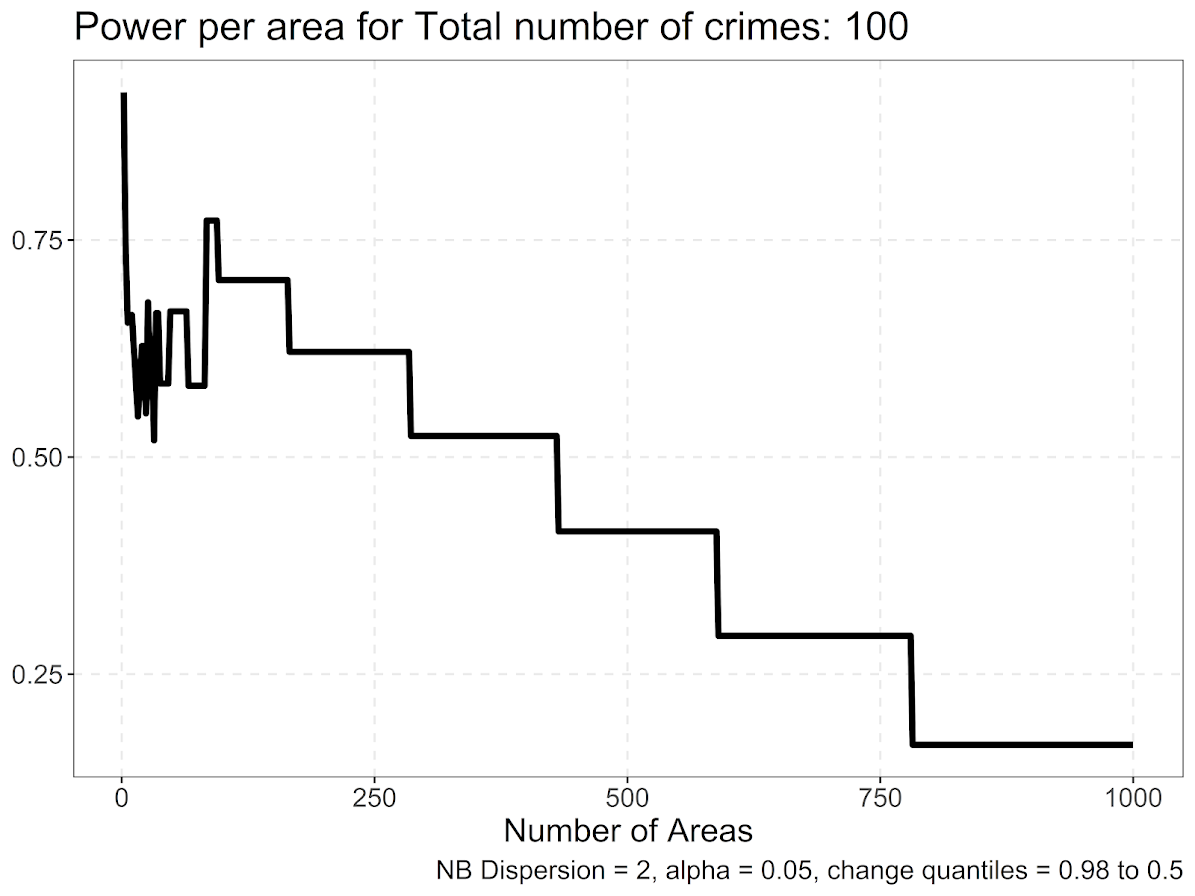So you can see here we never have very high power over this set of parameters. It is also non-monotonic and volatile at very small numbers of spatial units of analysis (at which the overdispersion assumption likely does not hold, and probably is not of much interest). But once that volatility tamps down we have stepped curve, that ends up happening to step whenever the original NB distribution changes from particular integer values.

So what happens with the power curve if we up the number of crimes to 3000?

``diff_suggest(3000)``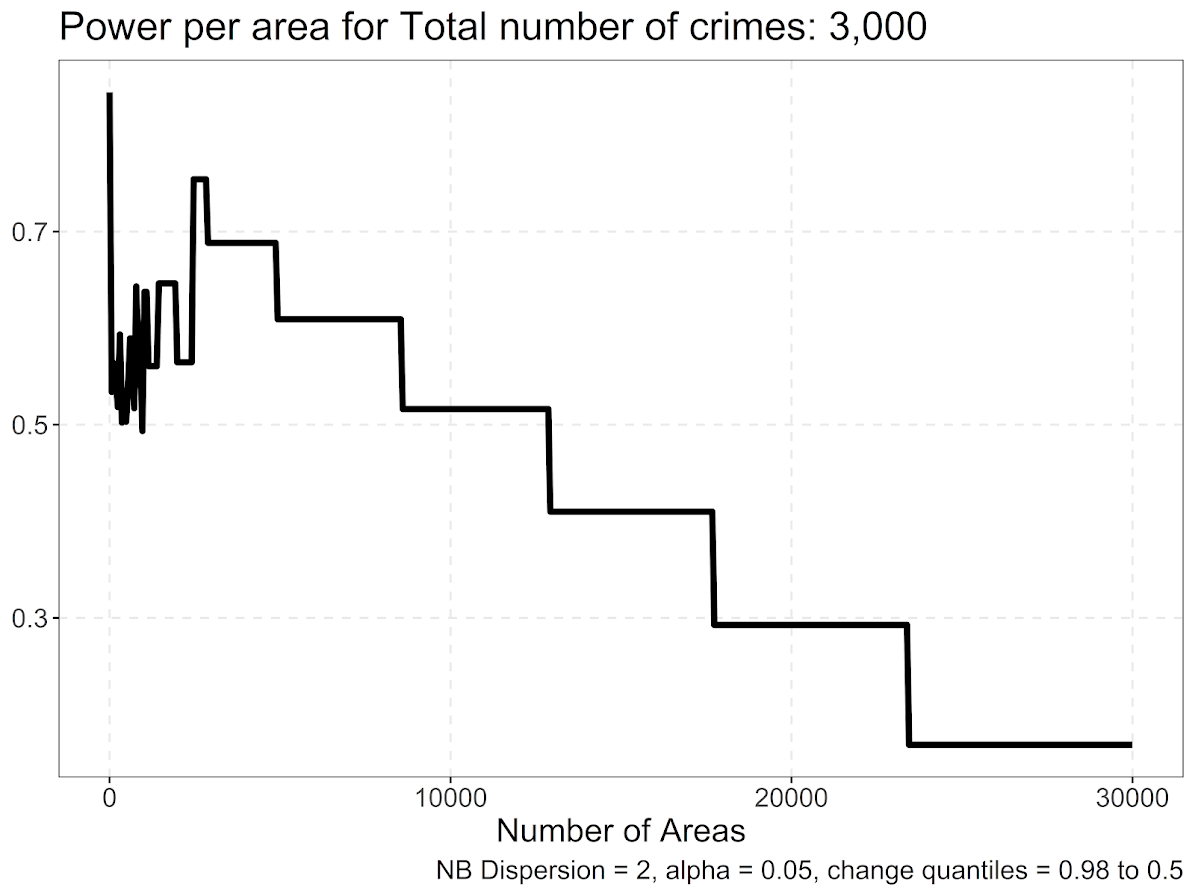So those two patterns are quite similar. It happens that when breaking down to the smaller units, the highest power scenario is when the crimes are subdivided into around 0.85 fewer spatial units than total crimes. So if you have 1000 crimes, in this scenario I would suggest to use 850 areas.

Also note the behavior when you break it down into a very large number of spatial units S, where `S >> N`, you get a progressive decline until around 0 power in this analysis. E.g. if you have 100 times more spatial units than observations, only a handful of locations have any crimes, and the rest are all 0’s. So you need to be able to tell the difference between 1/N and 0, which is tough (and any inferences you do make will just pretty much be indistinguishable from noise).

What about if we change the quantiles we are examining, and instead of looking at the very high crime place to the median, look at the 80th percentile to the 20th percentile:

``diff_suggest(3000, change_quant=c(0.8,0.2))``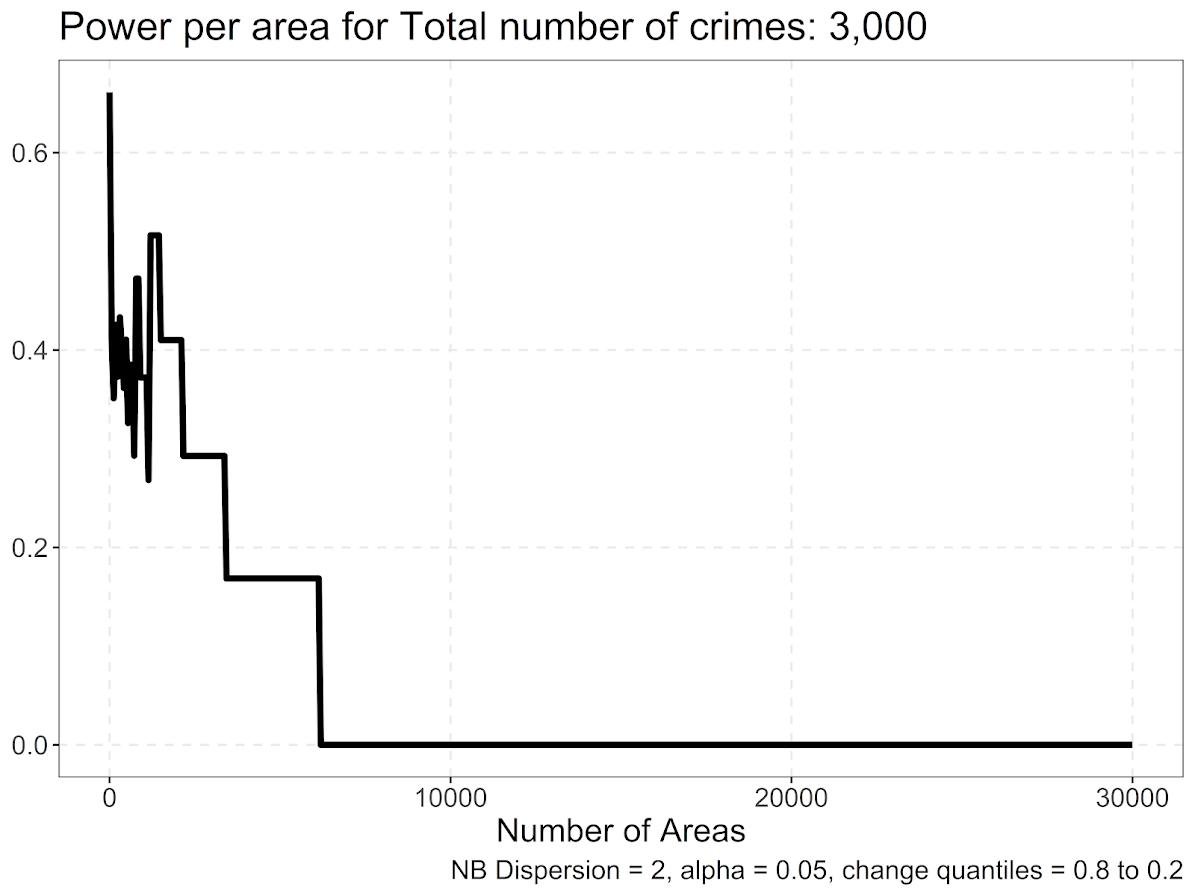We have a similar step pattern, but here the power is never as high as before, and only maxes out slightly above 0.5. It happens that this 0.5 power is around `number of crimes*0.45`. So this suggests that to uncover more middling transitions, one would need to have less than half the number of spatial units of crime observed. E.g. if you have 1000 crimes, I would not suggest any more than 450 spatial units of analysis.

So the first scenario, `crimes*0.85` you could say something like this is the highest power scenario to detect changes from very high crime locations (aka hot spots), to the middle of the distribution. For the second scenario (my preferred offhand), is to say `crimes*0.45` total spatial units results in the highest power scenario to detect more mild changes in the middle of the distribution (and detecting changes from hot spots to cold spots thus have even more power).

For now that is the best advice I can give for determining the spatial sample size for the SPPT test. Will have a follow up blog post on using R to make a grid to conduct the test.

Also I have been wondering about the best way to quantify changes in the overall ranking. I have not come upon a great solution I am happy with though, so will need to think about it some more.